Next: 3D Structure Model Up: Representation Previous: Representation

### Internal Geometry and Projection Model

In standard perspective projection, the mapping from a 3D coordinate onto the image plane is accomplished via the projection Equation 11.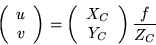(11)

However, we instead use the central projection representation as depicted in Figure 7. Here, the coordinate system's origin is fixed at the image plane instead of at the center of projection (COP). In addition, the focal length is parameterized by its inverse,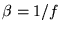. This camera model has long been used in the photogrammetry community and has also been adopted by Szeliski and Kang  in their nonlinear least squares formulation. The projection equation thus becomes Equation 12.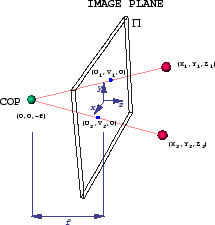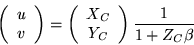(12)

Note how this projection decouples the camera focal length (f) from the depth of the point (ZC). In the traditional projection Equation 11, if ZC is fixed and the f is altered, the imaging geometry remains the same while the scale of the image changes. In other words, the cone of perspective rays remains fixed while the focal plane (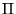) translates along the optical (Z) axis. We note that in the standard projection model, the imaging geometry (i.e. the perspective rays) are only altered by varying depth ZC which is the only way to alter the imaging geometry. Thus, f only acts as a scaling factor and the imaging geometry and the depth are encoded in ZC.

In our representation, however, the inverse focal length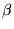alters the imaging geometry independently of the depth value ZC. State variable decoupling is known to be critical in Kalman filtering frameworks and is applicable here since we plan on putting both camera internal geometryand structure ZC into the internal hidden state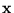.

Another critical property ofas opposed to f is that it does not exhibit numerical ill-conditioning. It can span the wide range of perspective projection but also the special case of orthographic projection which occurs when we set the focal length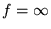and all rays project orthogonally onto the image plane. However, under orthographic projection,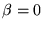which does not 'blow up' and maintains numerical stability in KF frameworks. We can thus combine both perspective and orthographic projection into the same so-called central projection framework without any numerical instabilities (this is demonstrated experimentally in the next section). This flexibility is not typical in many traditional computer vision approaches where perspective and orthographic projection must be treated quite differently. We now begin building our internal state vector with this well-behaved parameter,as in Equation 13.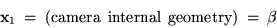(13)Next: 3D Structure Model Up: Representation Previous: Representation

1999-05-17